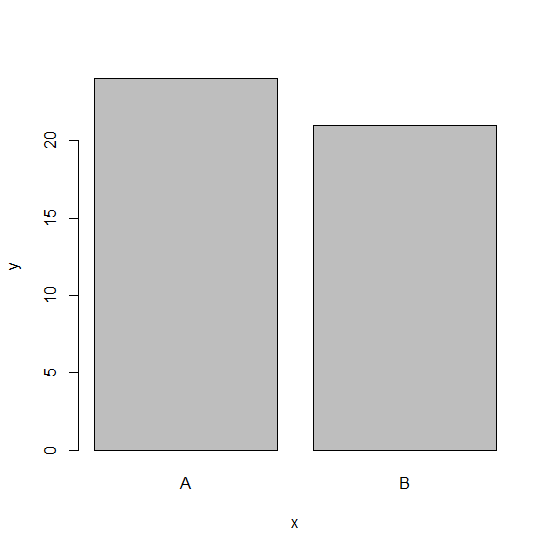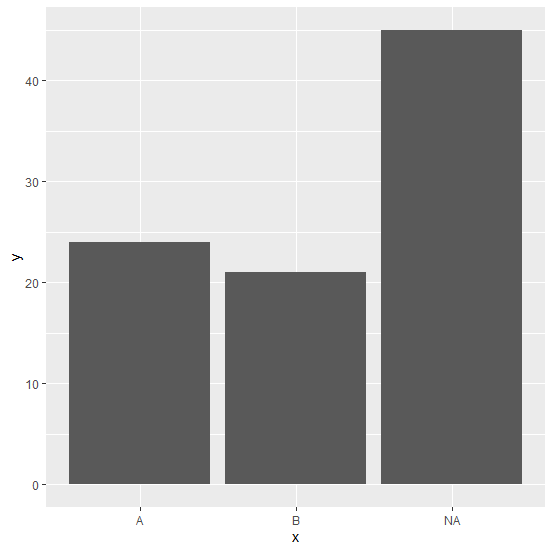# How to create a bar plot with bars for missing values in R?

To create a bar plot in R, we can use barplot function but if there exist some missing values in the data then we can use ggplot2 package. For example, if we have a data frame having two vectors say x and y, x containing categorical values with NA as one of the values and y having counts/frequency for each of the categories then the bar plot will be created by using the command ggplot(df,aes(x,y))+geom_bar(stat="identity").

## Example

Consider the below data frame −

Live Demo

> x<-c("A","B",NA)
> y<-c(24,21,45)
> df<-data.frame(x,y)
> df

## Output

     x  y
1    A 24
2    B 21
3 <NA> 45

Creating bar plot using barplot function −

> barplot(y~x,data=df)

## OutputLoading ggplot2 package and creating bar plot using geom_bar function of ggplot2 package −

> library(ggplot2)
> ggplot(df,aes(x,y))+geom_bar(stat="identity")

## Output